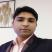# Conic Sections: Overview, Questions, Preparation

Conic Sections 2021 ( Conic Sections )

2.2K Views
This chapter has multiple topics listed below:Rachit Kumar SaxenaManager-Editorial

1. What are Conic Sections?
2. Weightage of Conic Sections
3. Illustrated Examples on Conic Sections
4. FAQs on Conic Section

## What are Conic Sections?

Geometric figures like Circle, Hyperbola, Parabola, and Ellipse are referred to as conic sections because they are formed due to the intersection of a plane and a cone.

### What is a Circle?

A circle is a figure in which every point is equidistant from the centre. The distance from the centre to any of its points is called a radius.

In general, a circle can be expressed by the following equation:

x2 + y2 + 2gx + 2fy + c = 0

Here, g, c, and f are constants, and the centre of the circle is (-g, -f). The radius of the circle here is r = square root of ( g2 + f2 - c).

If a circle passes through an origin then the equation becomes x2 + y2 + 2gx + 2fy.

### What is a Parabola?

A parabola is a curve-like figure on which any point is equidistant from a fixed point called focus and a straight line that is also fixed called the directrix.

A parabola has 4 forms viz. y2 = 4ax, y2 = -4ax, x2 = 4ay, and x2 = -4ay.

### What is an Ellipse?

An ellipse looks like a skewed circle and is referred to as a point set wherein the sum of all the points remains constant from 2 fixed points. The standard forms of an ellipse are given below:

(x2/a2) + (y2/b2) = 1 and (x2/b2) + (y2/a2) = 1.

In both these forms, a > b and b2 = a2(1 - e2) where e > 1.

### What is a Hyperbola?

A hyperbola is an open curve obtained from the intersection of the circular conic section with a plane. Here, the ratio of the distance of the points remains constant from a point called focus and a line called the directrix. The standard forms of Hyperbola are given below:

(x2/a2) - (y2/b2) = 1 and (y2/a2) - (x2/b2) = 1.

## Weightage of Conic Sections

All the conic sections' topics are extensively covered in Class XI and carry a weightage of 4 to 7 marks. It includes MCQ (Multiple Choice Questions), fill in the blanks, short and long answer questions.

## Illustrated Examples on Conic Sections

1. Calculate the equation of the circle with the centre at (0, 3) and radius 2.

Solution. The equation of the circle will be (x - 0)2 + (y - 3)2 = (2)2

x2 + y2 - 4y + 4 = 4

x2 + y2- 4y = 0.

2. The equation of the parabola is y2 = 20x. Find its focus, latus rectum's length, its axis and equation of the directrix.

Solution. From the general equation y2 = 4ax we get a = 5. Therefore, the length of the latus rectum will be 4a = 4 x 5 = 20.

The coordinate of the focus will be (5, 0), and the equation of the directrix will be x = -5.

The parabola's axis will be y = 0.

3. Find the equation of the ellipse with the centre at the origin and major axis falling on the y-axis going through points (3, 2) and (1, 6).

Solution. The centre is at (0, 0) and the equation of this ellipse is of the below form:

(x2/b2) + (y2/a2) = 1

As it passes through the points (3, 2) and (1, 6) this equation can be written as:

(9/b2) + (4/a2) = 1

Therefore, (1/b2) + (36/a2) = 1

From the above equations we get the values a2 = 40b2 = 10

Therefore, finally the equation becomes,

(x2/10) + (y2/40) = 1.

## FAQs on Conic Section

Q: What are the applications of conic sections?

A: Conic sections prove useful while studying 3D Geometry with numerous applications in electronics, architecture, and other fields.

Q: Is every circle an ellipse?

A: Yes, because a circle is a special case of an ellipse with the same distance from the centre for all the points.

Q: Give a real-life example of an ellipse.

A: The route in which the Earth travels around the Sun is elliptical.

Q: Give a real-life example of a hyperbola.

A: An hourglass looks like two hyperbolas next to each other if we ignore its neck.

Q: Give a real-life example of a parabola.

A: When the water erupts from a fountain and falls back into the pond, it follows a parabolic path.